Electron. J. Diff. Eqns., Vol. 1995(1995), No. 16, pp 1-49.

### Interfacial dynamics for thermodynamically consistent phase-field models with nonconserved order parameter Paul C. Fife & Oliver Penrose

Abstract:
We study certain approximate solutions of a system of equations formulated in an earlier paper (Physica D 43, 44-62 (1990)) which in dimensionless form are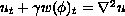,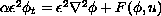,
whereis (dimensionless) temperature,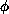is an order parameter,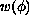is the temperature-independent part of the energy density, and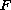involves the-derivative of the free-energy density. The constantsand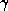are of order 1 or smaller, whereascould be as small as 10-8. Assuming that a solution has two single-phase regions separated by a moving phase boundary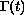, we obtain the differential equations and boundary conditions satisfied by the outer'solution valid in the sense of formal asymptotics away from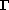and the inner' solution valid close to. Both first and second order transitions are treated. In the former case, the `outer' solution obeys a free boundary problem for the heat equations with a Stefan-like condition expressing conservation of energy at the interface and another condition relating the velocity of the interface to its curvature, the surface tension and the local temperature. There are O() effects not present in the standard phase-field model, e.g. a correction to the Stefan condition due to stretching of the interface. For second-order transitions, the main new effect is a term proportional to the temperature gradient in the equation for the interfacial velocity. This effect is related to the dependence of surface tension on temperature.

We also consider some cases in which the temperatureis very small, and possiblyoras well; these lead to further free boundary problems, which have already been noted for the standard phase-field model, but which are now given a different interpretation and derivation.

Finally, we consider two cases going beyond the formulation in the above equations. In one, the thermal conductivity is enhanced (to order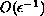) within the interface, leading to an extra term in the Stefan condition proportional (in two dimensions) to the second derivative of curvature with respect to arc length. In the other, the order parameter has m components, leading naturally to anisotropies in the interface conditions.

Submitted September 26, 1995. Published November 27, 1995. Math Subject Classification: 35K55, 80A22, 35C20.
Key Words: phase transitions, phase field equations, order parameter free boundary problems, interior layers.

Show me the PDF file (392 KB), TEX file, and other files for this article.

Paul C. Fife
Mathematics Department, University of Utah,
Salt Lake City, UT 84112, USA